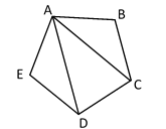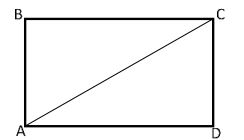# MA.912.GR.1.2Export Print
Prove triangle congruence or similarity using Side-Side-Side, Side-Angle-Side, Angle-Side-Angle, Angle-Angle-Side, Angle-Angle and Hypotenuse-Leg.

### Clarifications

Clarification 1: Instruction includes constructing two-column proofs, pictorial proofs, paragraph and narrative proofs, flow chart proofs or informal proofs.

Clarification 2: Instruction focuses on helping a student choose a method they can use reliably.

General Information
Subject Area: Mathematics (B.E.S.T.)
Strand: Geometric Reasoning
Status: State Board Approved

## Benchmark Instructional Guide

### Terms from the K-12 Glossary

• Angle
• Congruent
• Corresponding Angles
• Hypotenuse
• Right Triangle
• Similarity
• Triangle
• Vertical Angles

### Vertical Alignment

Previous Benchmarks

Next Benchmarks

### Purpose and Instructional Strategies

In grade 8, students were introduced to the concepts of congruence and similarity using transformations and proportional relationships. In Geometry, students prove triangle congruence or similarity using Side-Side-Side (SSS), Side-Angle-Side (SAS), Angle-Side-Angle (ASA), Angle-Angle-Side (AAS), Angle-Angle (AA) and Hypotenuse-Leg (HL) criteria. In later courses, these congruence and similarity criteria are critical in understanding applications of vectors and trigonometry.
• Students should develop the understanding of which criteria can be used to prove congruence and which criteria should be used to prove similarity.
• Criteria that can be used to prove congruence are SSS, SAS, ASA, AAS and HL, where the assumption is that the respective sides in the criterion are equal in length, and the respective angles are equal in measure.
• Criteria that can be used to prove similarity are AA, SAS and SSS, where the assumption is that the respective sides in the criterion are proportional in length with the same constant of proportionality, and the respective angles are equal in measure.
• Instruction includes developing the understanding that congruence implies similarity. Students should realize that if they have proved congruence of two triangles, then they have also proved similarity of the two triangles.
• Instruction includes the connection to the Logic and Discrete Theory benchmarks when developing proofs. Additionally, with the construction of proofs, instruction reinforces the Properties of Operations, Equality and Inequality. (MTR.5.1)
• Instruction utilizes different ways students can organize their reasoning by constructing various proofs when proving geometric statements. It is important to explain the terms statements and reasons, their roles in a geometric proof, and how they must correspond to each other. Regardless of the style, a geometric proof is a carefully written argument that begins with known facts, proceeds from there through a series of logical deductions, and ends with the statement you are trying to prove. (MTR.2.1
• For examples of different types of proofs, please see MA.912.LT.4.8
• Instruction includes the use of hatch marks, hash marks, arc marks or tick marks, a form of mathematical notation, to represent segments of equal length or angles of equal measure in diagrams and images.
• Students should understand the difference between congruent and equal. If two segments are congruent (i.e., PQMN), then they have equivalent lengths (i.e., PQ = MN) and the converse is true. If two angles are congruent (i.e., ∠ABC ≅ ∠PQR), then they have equivalent angle measure (i.e., $m$ABC = $m$PQR) and the converse is true.
• Problem types include mathematical or real-world context where students identify which one of the congruence or similarity criteria can be applied in specific cases; deduce information (e.g., vertical angles are congruent, reflexive property for a shared side or angle, corresponding angles when two parallel lines are cut by a transversal are congruent) from given images to determine the congruence or similarity criterion needed to prove triangle congruence or similarity; and determine what piece of information about a pair of triangles must be added to prove triangles are congruent or similar by a certain criterion. (MTR.6.1
• Instruction includes exploring why Hypotenuse-Leg (HL) can be used to show right triangles are congruent. Students should be able to realize that HL is a specific case of Side-Side-Side (SSS) and Side-Angle-Side (SAS) applying the Pythagorean Theorem.
• Instruction for some theorems, relationships or postulates may be necessary in order to prove the validity of congruence or similarity criteria.
• For example, to prove the validity of the AA similarity criterion, students will need knowledge of the Triangle Sum Theorem.

### Common Misconceptions or Errors

• Students may confuse the congruence and similarity versions of the Side-Side-Side and Side-Angle-Side criteria. To address this misconception, provide students with counterexamples and opportunities to discuss the difference.
• Students may try to use Angle-Angle, Angle-Angle-Angle or Side-Side-Angle to prove congruence

• Pentagon ABCDE, as shown below, is a regular pentagon.• Part A. Can you identify two possible congruent triangles in the figure?
• Part B. Write a congruence statement for the two triangles that are congruent.
• Part C. What theorem or postulate can be used to prove the two triangles congruent?
• Part D. Prove that the two triangles chosen in Part A are congruent to one another.
• Part E. Determine a triangle that is congruent to triangle ACD.
• Part F. Repeat Parts B through D with the new pair of triangles.

• Part A. Draw a triangle with side lengths 6 inches, 7 inches and 10 inches. Compare your triangle with a partner.
• Part B. Draw a triangle with side lengths 4 inches and 6 inches, and with a 70° angle in between those side lengths. Compare your triangle with a partner.
• Part C. Draw a triangle with angle measures of 40° and 60°, and a side length of 5 inches between those angle measures. Compare your triangle with a partner.
• Part D. Based on the comparison of triangles created from Parts A, B and C, what can you conclude about criteria for determining triangle congruence?

### Instructional Items

Instructional Item 1
• Use rectangle ABCD to fill in the blanks.In a rectangle opposite sides are _______ which means ¯ ≅ ¯ . Triangles ABC and CDA can be proven congruent by Hypotenuse-Leg because _____ is the hypotenuse for both triangles.

*The strategies, tasks and items included in the B1G-M are examples and should not be considered comprehensive.

## Related Courses

This benchmark is part of these courses.
1200400: Foundational Skills in Mathematics 9-12 (Specifically in versions: 2014 - 2015, 2015 - 2022, 2022 and beyond (current))
1206310: Geometry (Specifically in versions: 2014 - 2015, 2015 - 2022, 2022 and beyond (current))
1206320: Geometry Honors (Specifically in versions: 2014 - 2015, 2015 - 2022, 2022 and beyond (current))
1206315: Geometry for Credit Recovery (Specifically in versions: 2014 - 2015, 2015 - 2022, 2022 and beyond (current))
7912065: Access Geometry (Specifically in versions: 2015 - 2022, 2022 and beyond (current))

## Related Access Points

Alternate version of this benchmark for students with significant cognitive disabilities.
MA.912.GR.1.AP.2: Identify the triangle congruence or similarity criteria; Side-Side-Side, Side-Angle-Side, Angle-Side-Angle, Angle-Angle-Side, Angle-Angle and Hypotenuse-Leg.

## Related Resources

Vetted resources educators can use to teach the concepts and skills in this benchmark.

## Formative Assessments

Justifying a Proof of the AA Similarity Theorem:

Students are asked to justify statements of a proof of the AA Similarity Theorem.

Type: Formative Assessment

Describe the AA Similarity Theorem:

Students are asked to describe the AA Similarity Theorem.

Type: Formative Assessment

What Is the Triangle Relationship?:

Students are asked to write an informal justification of the AA Similarity Theorem.

Type: Formative Assessment

Drawing Triangles SSA:

Students are asked to draw a triangle given the lengths of two of its sides and the measure of a nonincluded angle and to decide if these conditions determine a unique triangle.

Type: Formative Assessment

Drawing Triangles SAS:

Students are asked to draw a triangle given the measures of two sides and their included angle and to explain if these conditions determine a unique triangle.

Type: Formative Assessment

Drawing Triangles ASA:

Students are asked to draw a triangle given the measures of two angles and their included side and to explain if these conditions determine a unique triangle.

Type: Formative Assessment

Drawing Triangles AAS:

Students are asked to draw a triangle given the measures of two angles and a non-included side and to explain if these conditions determine a unique triangle.

Type: Formative Assessment

Drawing Triangles AAA:

Students are asked to draw a triangle with given angle measures, and explain if these conditions determine a unique triangle.

Type: Formative Assessment

Similar Triangles - 2:

Students are asked to locate a pair of similar triangles in a diagram, explain why they are similar, and use the similarity to find an unknown length in the diagram.

Type: Formative Assessment

Similar Triangles - 1:

Students are asked locate a pair of similar triangles in a diagram, explain why they are similar, and use the similarity to find two unknown lengths in the diagram.

Type: Formative Assessment

## MFAS Formative Assessments

Describe the AA Similarity Theorem:

Students are asked to describe the AA Similarity Theorem.

Drawing Triangles AAA:

Students are asked to draw a triangle with given angle measures, and explain if these conditions determine a unique triangle.

Drawing Triangles AAS:

Students are asked to draw a triangle given the measures of two angles and a non-included side and to explain if these conditions determine a unique triangle.

Drawing Triangles ASA:

Students are asked to draw a triangle given the measures of two angles and their included side and to explain if these conditions determine a unique triangle.

Drawing Triangles SAS:

Students are asked to draw a triangle given the measures of two sides and their included angle and to explain if these conditions determine a unique triangle.

Drawing Triangles SSA:

Students are asked to draw a triangle given the lengths of two of its sides and the measure of a nonincluded angle and to decide if these conditions determine a unique triangle.

Justifying a Proof of the AA Similarity Theorem:

Students are asked to justify statements of a proof of the AA Similarity Theorem.

Similar Triangles - 1:

Students are asked locate a pair of similar triangles in a diagram, explain why they are similar, and use the similarity to find two unknown lengths in the diagram.

Similar Triangles - 2:

Students are asked to locate a pair of similar triangles in a diagram, explain why they are similar, and use the similarity to find an unknown length in the diagram.

What Is the Triangle Relationship?:

Students are asked to write an informal justification of the AA Similarity Theorem.

## Student Resources

Vetted resources students can use to learn the concepts and skills in this benchmark.

## Parent Resources

Vetted resources caregivers can use to help students learn the concepts and skills in this benchmark.